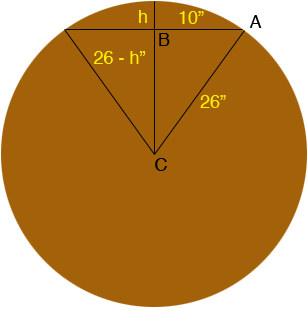SEARCH HOMEMath Central Quandaries & QueriesQuestion from Bob: I have a circle with a 26" radius and a cord whose length is 20". How can I find the height of the arc at the center point of the cord?Hi Bob,

In the diagram below h is the height of the arc at the centre point of the cord in inches and C is the centre of the circle.Since triangle ABC is a right triangle you can use Pythagoras' Theorem to obtain

102 + (26 - h)2 = 262

Expanding yields

100 + 262 - 52h + h2 = 262 or
h2 - 52h + 100 = 0

Solving using the general quadratic yields h = 2 inches or h = 50 inches.(50 inches is the distance from B to the opposite side of the circle.)

PennyMath Central is supported by the University of Regina and The Pacific Institute for the Mathematical Sciences.